# MySQL查询where条件的顺序对查询效率的影响

where条件的运行是从右到左的，将选择性强的条件放到最右边，可以先过滤掉大部分的数据（而选择性不强的条件过滤后的结果集仍然很大），在根据其它条件过滤时，需要比较的数据量就少，提高查询的效率。

## 实践是检验真理的唯一标准

### 单列的辅助索引

#### 1. name列有索引

EXPLAIN
SELECT id,name,age from test_condition where name='test99500' and age=30EXPLAIN
SELECT id,name,age from test_condition where age=30 and name='test99500'#### 2. name列有索引，age列有索引

EXPLAIN
SELECT id,name,age from test_condition where name='test99500' and age=30EXPLAIN
SELECT id,name,age from test_condition where age=30 and name='test99500'### 多列的联合索引

#### 联合索引<name,age>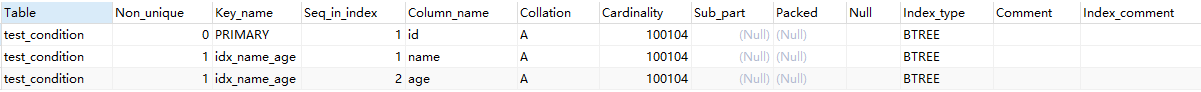#### 1. 联合索引的列都使用

EXPLAIN SELECT * from test_condition where name='test100' and age = 30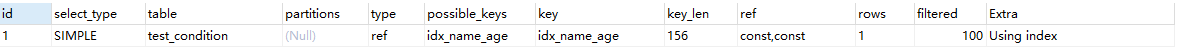EXPLAIN SELECT * from test_condition where age = 30 and name='test100'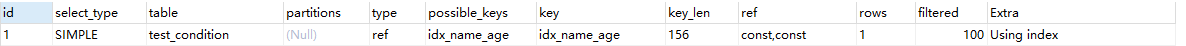#### 2. 仅使用联合索引中的一列

EXPLAIN SELECT * from test_condition where name='test100'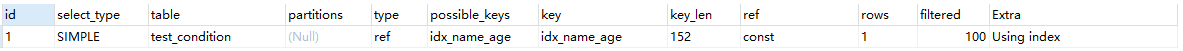EXPLAIN SELECT * from test_condition where age = 30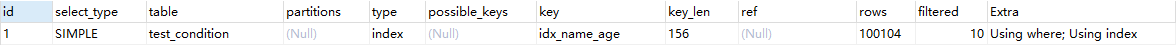EXPLAIN SELECT * from test_condition where name='test100'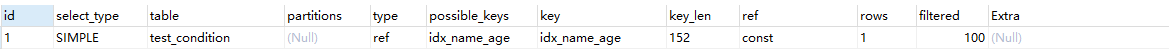EXPLAIN SELECT * from test_condition where age = 30posted @ 2017-09-07 13:39  Acode  阅读(29088)  评论(3编辑  收藏  举报